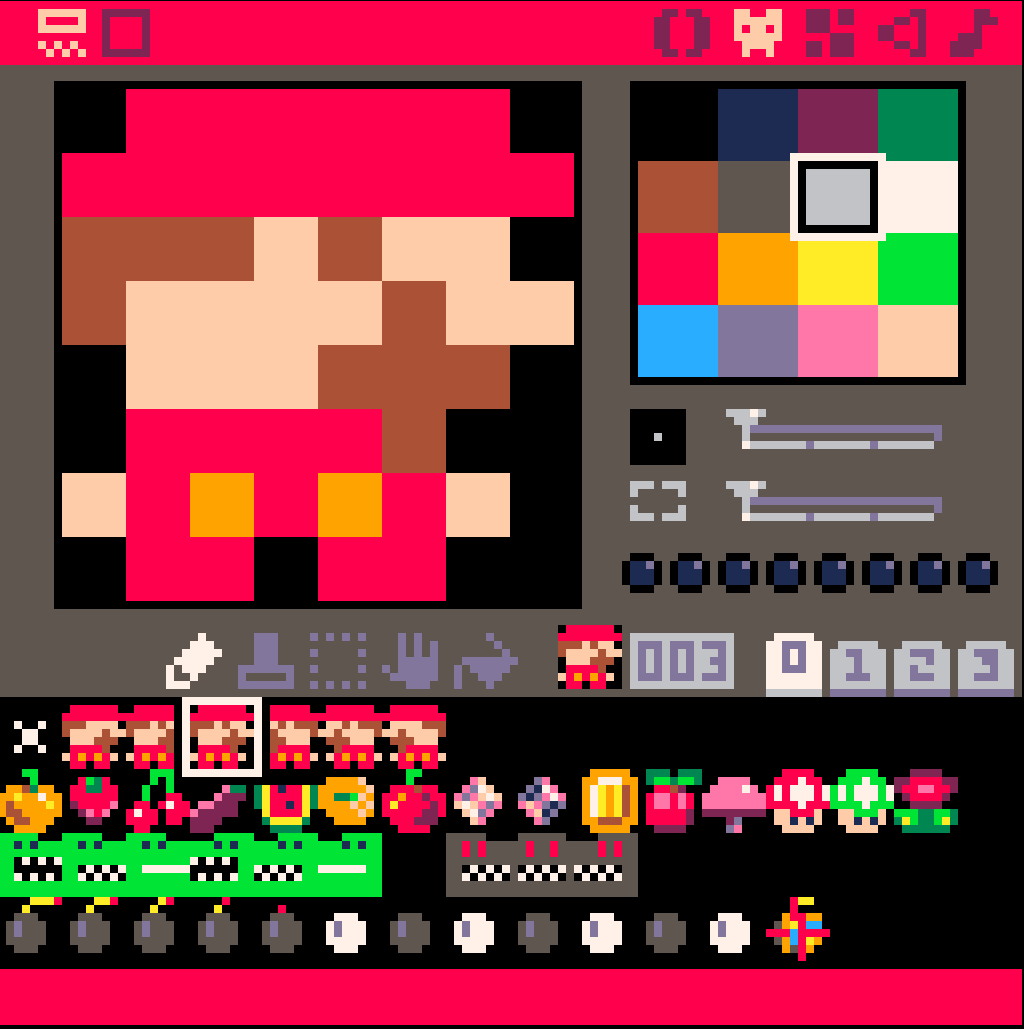Log InFollowpeymanx

Hey, I am PEYMANx

 paddle-xpeymanx2:: Unfold ::2Ok,
This is my second game...
Pico8 is reallllly fun## Source code at Github:

🤩 HAVE FUN

P#119259 2022-10-18 18:58 ( Edited 2022-10-24 06:35)

 Mario vs Khabis 🎮peymanx:: Unfold ::Cart #mario_vs_khabis-1 | 2022-10-22 | Code ▽ | License: CC4-BY-NC-SAWhat's up guys
I made a video to explain how Lua works - in Persian
and this game is the result
only in 20min you can make a wonderful game

In Persian "khabis" means bad guy.
Khabis and mario try to get fruits and avoid bombs.
a simple senario and addictive game :)Collision Detection
In physics, a collision is any event in which two or more bodies exert forces on each other in a relatively short time.```function collision(a,b,x,y) if (a <= x and a+8 >=x or x <= a and x+8 >=a) and (b <= y and b+8 >=y or y <= b and y+8 >=b) then return true end return false end```

# Mario vs Khabis

This game is part of a tutorial

Github source code:
https://github.com/peymanx/Mario-vs-Khabis[8x8]
vs[8x8]

P#119095 2022-10-14 12:30 ( Edited 2022-10-26 10:20)

 peyman-x puzzle hitterpeymanx2:: Unfold ::Cart #px_puzzle-1 | 2022-10-14 | Code ▽ | License: CC4-BY-NC-SA2First I load my image to a table,
final positions in x,y and random position in (a,b)

 ```function load_image() --load the image me = {} for j=0,8 do row={} for i=0,8 do add(row,{ spr=j*16+i, x=i*8+30, y=j*8+20, a=rnd(150), b=130, }) end add(me, row) end end```

then I call a magnet() function to move a=>x and b=>y

 ```function magnet() local step=1 for j=1,8 do for i=1,8 do local p = me[j][i] x= p.a y= p.b if p.a > p.x then p.a-=step end if p.a < p.x then p.a+=step end if p.b > p.y then p.b-=step end if p.b < p.y then p.b+=step end end```

## Happy Coding

P#119089 2022-10-14 09:11

Generated 2023-02-08 10:38:10 | 0.056s | Q:17